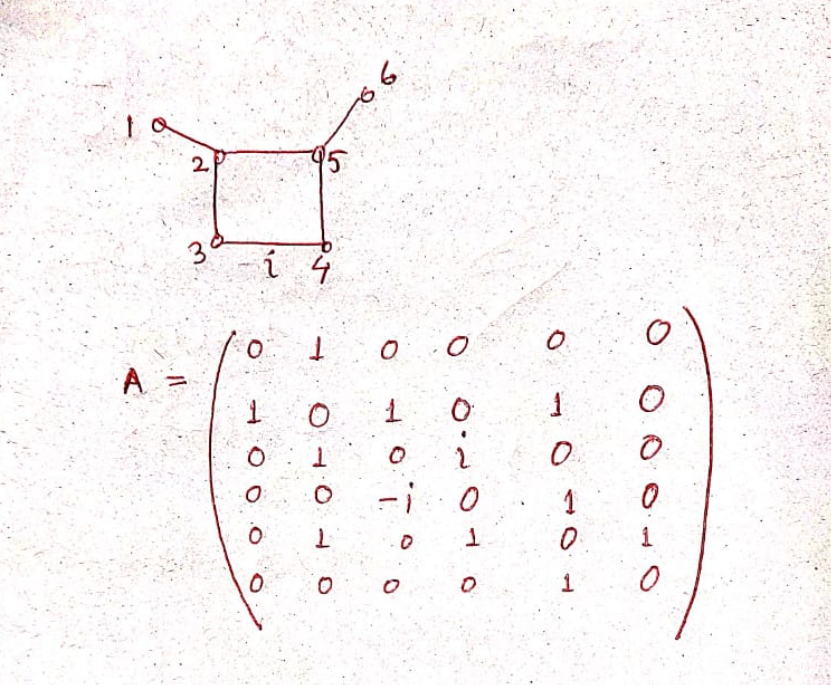# Revision history [back]

### How to construct the class of all connected weighted unicyclic graphs on $n$ vertices, where exactly one edge of the cycle in the unicyclic graphs

How to construct the class of all connected weighted unicyclic graphs on $n$ vertices, where exactly one edge of the cycle in the unicyclic graphs has weight $i\,(=\sqrt{-1})$ and remaining all the edges in the graphs are of weight $1$ For example consider the following graph together with its adjacency matrix. In the adjacency matrix we take the entry (3,4) as $i$ and $(4,3)$ as $-i$, that is, just the conjugate of $i$

### How to construct the class of all connected weighted unicyclic graphs on $n$ vertices, where exactly one edge of the cycle in the unicyclic graphs

How to construct the class of all connected weighted unicyclic graphs on $n$ vertices, where exactly one edge of the cycle in the unicyclic graphs has weight $i\,(=\sqrt{-1})$ and remaining all the edges in the graphs are of weight $1$ For example consider the following graph together with its adjacency matrix. In the adjacency matrix we take the entry (3,4) as $i$ and $(4,3)$ as $-i$, that is, just the conjugate of $i$

### How to construct the class of all connected weighted unicyclic graphs on $n$ vertices, where exactly one edge of the cycle in the unicyclic graphs

How to construct the class of all connected weighted unicyclic graphs on $n$ vertices, where exactly one edge of the cycle in the unicyclic graphs has weight $i\,(=\sqrt{-1})$ and remaining all the edges in the graphs are of weight $1$ For example consider the following graph together with its adjacency matrix. In the adjacency matrix we take the entry (3,4) as $i$ and $(4,3)$ as $-i$, that is, just the conjugate of $i$### How to construct the class of all connected weighted unicyclic graphs on $n$ vertices, where exactly one edge of the cycle in the unicyclic graphs

How to construct the class of all connected weighted unicyclic graphs on $n$ vertices, where exactly one edge of the cycle in the unicyclic graphs has weight $i\,(=\sqrt{-1})$ and remaining all the edges in the graphs are of weight $1$ For example consider the following graph together with its adjacency matrix. In the adjacency matrix we take the entry (3,4) as $i$ and $(4,3)$ as $-i$, that is, just the conjugate of $i$### How to construct the class of all connected weighted unicyclic graphs on $n$ vertices, where exactly one edge of the cycle in the unicyclic graphs

How to construct the class of all connected weighted unicyclic graphs (a connected graph on $n$ vertices is said to be unicyclic if it has $n$ edges) on $n$ vertices, where exactly one edge of the cycle in the unicyclic graphs has weight $i\,(=\sqrt{-1})$ and remaining all the edges in the graphs are of weight $1$ For example consider the following graph together with its adjacency matrix. In the adjacency matrix we take the entry (3,4) as $i$ and $(4,3)$ as $-i$, that is, just the conjugate of $i$